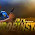## How to

When solving these problems, learning by doing is much better than learning by reading. I encourage to you read only as far in the solution as you need, then trying to solve the problem. If you get stuck, try reading a little further. And of course, let me know if you find a better solution!

## Friday, February 28, 2014

### Problem:  Write a function which returns the square root of an integer.  Return an integer which is the floor of the integer closest to the value.  Implement an algorithm with efficiency better than O(sqrt(n))

int sqrtFlr(int x) {...}

In the last post, we implemented a solution to this problem, which was O(log(n)).

The solution was:
1.  Create a ceiling and floor, where the floor is 0 and the ceiling is the number.
2.  Try the square of halfway between the ceiling and floor.
3.  Adjust the ceiling or floor based up on the result.
4.  Continue 2 and 3 and return the floor when the ceiling is one more than the floor.

Now, let's implement this is code:

int sqrtFlr(int x) {

int floor = 0;
int ceiling = x;
int halfway;

while (floor != (ceiling - 1)) {
halfway = floor + (ceiling - floor) / 2);
if ((halfway * halfway) > x) {  // halfway is the ceiling!
ceiling = halfway;
} else if ((halfway * halfway < x)) {//halfway is the floor!
floor = halfway;
else {   //we have a perfect square
return halfway;
}

return floor;
}

Let's try an example now, say 284.

When we start:
floor = 0
celing = 284.

we enter the while loop.
Now, halfway is 142.
142^2 is much bigger than 284, so we get:
floor = 0;
ceiling = 142

continuing through the while loop:
floor = 0;
ceiling = 142;
halfway = 71;
71^2 is still much bigger than 284, so we get:
floor = 0;
ceiling = 71;

staying in the while loop, we continue:
floor = 0;
ceiling = 71;
halfway = 35;  (35^2 is much bigger still)

staying in the while loop, we continue:
floor = 0;
ceiling = 35;
halfway = 17;  (17^2 is much bigger still)

staying in the while loop, we continue:
floor = 0;
ceiling = 17;
halfway = 8;  (8^2 = 64, which is smaller)

staying in the while loop, we continue:
floor = 8;
ceiling = 17;
halfway = 12;  (12^2 = 144, which is smaller)

staying in the while loop, we continue:
floor = 12;
ceiling = 17;
halfway = 14;  (14^2 = 196, which is smaller)

staying in the while loop, we continue:
floor = 14;
ceiling = 17;
halfway = 15;  (15^2 = 225, which is smaller)

staying in the while loop, we continue:
floor = 15;
ceiling = 17;
halfway = 16;  (16^2 = 256, which is smaller)  and we set floor to 16.

Now, when we go to the while loop, it fails and we fall out, and return 16.

16 is the correct answer here.

So, this solution seems to work.

Let's think of edge cases here.  Try a perfect square, say 16.
floor = 0;
ceiling = 16;
halfway = 8;  (8^2 = 64, which is larger).

Continuing through the for loop:
floor = 0;
ceiling = 8;
halfway = 4;  (4^2 = 16, which is equal, trigger the else in the if statement, and returns true, so this works).

Ok-- any edge cases.  Well, we could try 0 and 1, and this works.  How about negative numbers.  Well, let's return -1 in this case, and then we're done.

int sqrtFlr(int x) {

int floor = 0;
int ceiling = x;
int halfway;

if (x < 0) return -1;  //error case.

while (floor != (ceiling - 1)) {
halfway = floor + (ceiling - floor) / 2);
if ((halfway * halfway) > x) {  // halfway is the ceiling!
ceiling = halfway;
} else if ((halfway * halfway < x)) {//halfway is the floor!
floor = halfway;
else {   //we have a perfect square
return halfway;
}

return floor;
}

And there is is.  An O(log n) solution to returning square root floor!

/* Please send along any bugs or optimizations! */

1.Nice! In computer science, we'd say this problem can be "reduced" to a Binary Search problem. Meaning, they're equivalent.

1.Very nice pick-up! Thanks Amin.

2.Nice, but doesn't work for x = 1, does it? It'll never enter the loop (because the floor is initially already one less than the ceiling), hence returning the initial floor, i.e. 0 ... so do we just make 1 a special case too?

1.Nice catch Amir! I just noticed that very same flaw in my own solution

3.I noticed that if x is larger than 100,000, then there is an overflow issue, so I am thinking is there a way to lower down that upperbound first....

4.Watch your favourtie pinoy channel replays and all the pinoy flix tv tambyan replays online in hd.

5.Get your favourite Filipino tv shows online and all Teleserye Pinoy Channel Tv Get videos and download all Pinoy tv